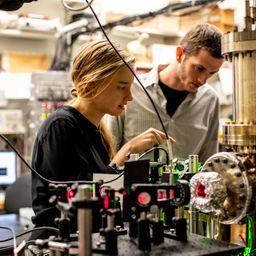## a. 2.82 \times 10^{-37} mb. 1.06 \times 10^{-32} Jc. 2.88 \times 10^{-35} \%

Quantum Physics

Atomic Physics

### Discussion

You must be signed in to discuss.
##### Top Physics 103 Educators##### Christina K.

Rutgers, The State University of New Jersey##### Marshall S.

University of Washington##### Aspen F.

University of Sheffield### Video Transcript

Okay, so for this problem, Chinese jumping from a window and the window is 50 meters above the ground. So let's call it that. D is 50.0 meters and then his master's 75 kilograms, 75 or 75.0 75.0. And the picture was taken at a time frame of five Bella seconds. And then first we want to know that I broke the wavelength when he's at the bottom so that the broccoli win playing CSIS found by evaluating Lambda equals each overpay. And then p is he got to m V. So we have his mass. But we don't know have his final velocity so we can find that using energy conservation. Um and so the system of the kid and then the, um or maybe the man and the, uh, Earth is a closed system. And so now there's no outside forces acting, so energy is conserved. Therefore, the change in potential energy is equal toe change in kinetic energy. So that means formulaic Lee the initial potential energy, the change of potential energy. MGH magazine, that is ah, equal toe One happened B squared. And then if you want to solve for B can Ah, cancel the ama's Bring the two to the other side and get the square root of two G h so that we can go back and update this formula and we can say it's equal to age. Right? Um, I am scared of to G. H. And, um, if we evaluate that actually, I did already. So I got two point 2.82 times 10 to the minus 37 meters. So pretty small way We always expect macroscopic objects to have, um to have no or, you know, normal classical objects to have very small to publicly blanks. Um, and the uncertainty. So if I answer to you, we want to use the energy time uncertainty relationship so we can say Delta E times, Delta T walls age divided by two times two pi. Not sure why I wrote it this way. I mean, I think I'm used to writing at his age bar, but I'm using a JJ. I've conventionally been using it. Um And so anyway, what we so we want to solve for adult eee so we can divide both sides by Delta T. So I wouldn't do that. So that comes over here. And then if I put that into a calculator which exploded earlier, actually made this video earlier and did not say that. So I get 1.5 times 10 to the minus 32 drools. And for a print. See, we want to get, um, what percent error? So we want to hire a daughter, he over a the kinetic energy at the bottom. But we know the kinetic energy is equal to the change in potential. Just kind of based what we talked about for a So this is gonna be mg and then plugging that into a calculator. I ended up getting 2.87 times, 10 to the minus 35% percent.University of Washington

#### Topics

Quantum Physics

Atomic Physics

##### Top Physics 103 Educators##### Christina K.

Rutgers, The State University of New Jersey##### Marshall S.

University of Washington##### Aspen F.

University of Sheffield